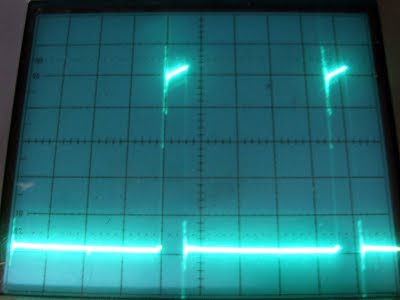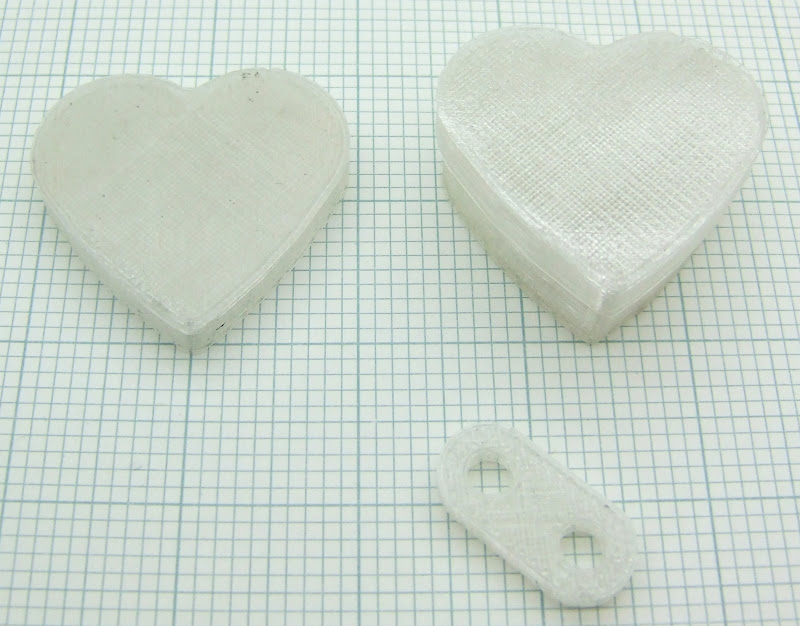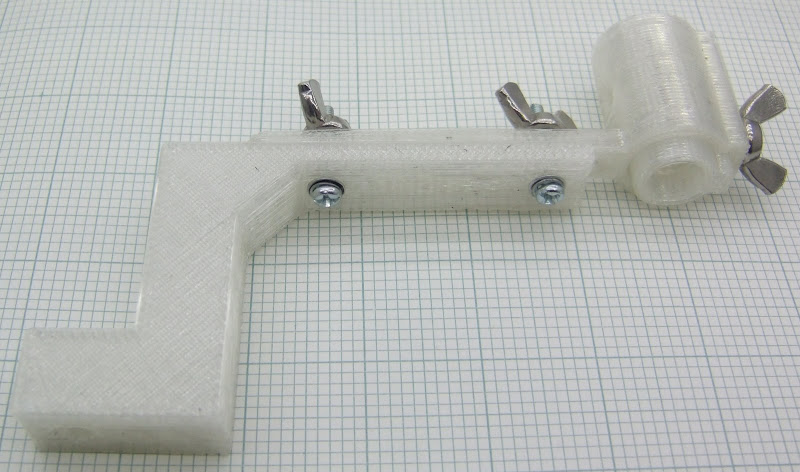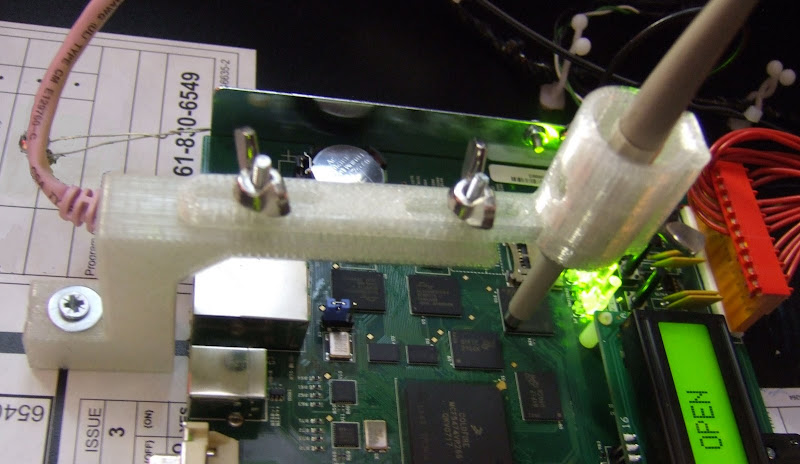## Sunday, 9 August 2009

### Mixed decay, mixed blessing

Having set the correct off time to suit my motor I can now micro step it with equal spaced steps, but only if I disable the mixed decay mode.When the chopper switches off it can do it two ways. It can turn on both low side transistors. That short circuits the motor and lets the current recirculate. If the coil was a perfect inductor and the transistors perfect switches, the current would circulate forever and you would have a superconducting magnet. Real coils and transistors have some resistance, which causes the current to decay, but as these are relatively small the mode is called slow decay.

This is fine and efficient until you take the motor's back emf into account. During the rising part of the sine wave the magnet is moving towards the pole piece, so it generates a voltage that causes the current to fall faster. The on time gets longer to compensate and all is well.

On the trailing edge of the sine curve the magnet has gone past the pole piece and generates a voltage that increases the current in the coil. If it is going fast enough it can mean that the current doesn't fall at all during the slow decay period. As I showed previously that can cause a severely distorted waveform which makes the motor noisy.The Allegro chips offer a mixed decay mode, where they switch to fast decay for part of the chopping cycle on the downward half of the sine curve. In fast decay mode one low side and one high side transistor turn on and reverse the voltage across the motor. That overcomes the BEMF and causes the current to fall much faster. It also returns current to the supply rail, which can upset some power supplies if there isn't some other load to absorb it.

Mixed decay gives a current waveform like this: -The off time is fixed, so the current falls further making the ripple greater. If you set the percentage fast decay to give a clean waveform at your top speed, then the ripple increases at slower speeds. It is maximum when stationary, when there is no BEMF and fast decay is not required at all.

The problem is that the target current is the trip point of the comparator, so it is the peak of the chopping waveform. That means the average current is less by half the ripple current giving a positional error.

With the low inductance motor I am using, the ripple current has a large amplitude, so the error introduced when the motor is stationary is about the same as a micro step. That means the first step with fast decay is about twice as big as it should be and the last step is virtually zero.

With the A3977 I can disable fast decay and the steps are fairly even, but fast running is then distorted. The PFD setting needs to change with speed.

With the A3983 that I have used on my new extruder controller the PFD setting is fixed at 31.25%. That means I can't get evenly spaced microsteps with the NEMA17's that I have, when running slowly. Not a big problem with the extruder because I plan to gear it down 40:1, which means one micro step is only about 0.02mm. I am only using microstepping to give smooth motion rather than extra resolution.

The problem is exaggerated because not only am I using a low inductance motor, but I am also trying to run it at 1A, whereas it is rated for 2.5A. At 2.5A the off time would be about 2.5 times smaller, so the ripple would be 2.5 times less. The steps in the current waveform would be 2.5 times bigger, so the distortion would be reduced by 6.25 times. As it is about one microstep now, it would reduce to 1/6th of a microstep, so would be acceptable. The temperature rise would then be 6.25 times greater of course.

I was planning to use A3977s for my axis control though, where positional accuracy is important. I am beginning to think I will be better off just using dual H-bridges and doing the rest in software using a powerful micro with a fast ADC.

To be able to cope with a wide variety of motors you need to change the current, the off time setting and the percentage of fast decay. You also need to take the ripple current amplitude into account to control the average current, rather than the peak. All these things could be automated with a software solution.

## Thursday, 6 August 2009

### Motor Maths

In a previous post I showed the pitfalls of constant off time choppers like the A3977. Basically you have to set the off time long enough to be able to deliver the lowest current step of the microstepping, otherwise the steps are not equally spaced.

Forrest raised the point of how do you do that without a scope. It is fairly easy to calculate from the target current, supply voltage and motor resistance using nothing more complex than Ohm's law. It did take me a few days to come up with a formula that matched my measurements, but that was because I was accidentally running the chip without synchronous rectification enabled.

The motor current is equal to the reference voltage divided by 8 times the sense resistor. The maximum sense voltage is 0.5V, so a sensible value for the sense resistors is 0.2Ω, giving 2.5A maximum with 4V at the reference pin. My lash up uses two 0.5Ω resistors in parallel giving a 2A maximum.

The minimum current required on the first step of the microstep will be I × sin(π/2n), where n is the number of microsteps. In this case n is 8 so the smallest current step is 19.5% of the full current. To calculate the minimum off time needed we need to be able to work out what the duty cycle will be to get a given current.

Here is what the sense resistor waveform looks like when the current is set to 1A and the motor is stationary. The on period is 3μS and the off period is 20μS. The supply voltage is 12V.The sloping top of the waveform is actually an exponential curve, but at this scale it is very close to linear and to simplify the calculations I have just used the average value.

So we know that 1A flows from the supply for every 3 out of 23μS. That gives an average current from the supply of 1A × 3 /23 = 130mA. Indeed the supply current measures 260mA as there are two coils energised (I set it to full step mode to make this measurement).

When the chopper is on energy flows into the inductance of the motor, increasing its magnetic field slightly. During the off time the current flows in a loop consisting of the motor and the two low side transistors. Power is dissipated by the motor's resistance, so it loses energy by its magnetic field decreasing slightly. We can calculate the duty cycle by reasoning that the energy going in during the on state must equal the energy coming out in the off state.

The motor is a Lin 4118S-62-07 NEMA17 motor I got from Makerbot. It has a coil resistance of only 0.8Ω. That means the resistance of the sense resistor and the on resistance of the FETs in the chip are significant in the calculation.

During the on state current flows through one top transistor, the coil, one bottom transistor and the sense resistor. All the resistances convert electricity to heat so the power going into the magnetic field is the power drawn from the supply minus the resistive losses in the circuit.Power = VI or I2R, Energy = PT.

So we have (Vsupply × I - I2 × (Rmotor + Rsense + RDS(on) source +RDS(on) sink)) × Ton.

In this example (12 - (0.8 + 0.25 + 0.36 + 0.45)) * 3 = 30.4 μJ.

During the off state the current flows through the motor resistance and two low side transistors, so the energy lost is: I2 × (Rmotor + 2 × RDS(on) sink) × Toff.

In this example (0.8 + 2 × 0.36) × 20 = 30.4 μJ, so theory matches practice (using typical values from the datasheet for RDS(on)), always very satisfying.

So if we call the total resistance in the circuit with the switch on Ron and the total when it is off Roff we have: -
Ron = Rmotor + Rsense + RDS(on) source +RDS(on) sink = 1.86Ω
Roff = Rmotor + 2 × RDS(on) sink = 1.52Ω

Then Toff = Ton (V/I - Ron) / Roff
For our example if we set the minimum Ton (Tblank) to be 1μS, I = 0.195A, so Toff is 39μS.

At 1A Ton will then be ~6μS. So the minimum chopping frequency will be ~22kHz and the maximum will be 25kHz.

CT = Tblank / 1400 = 714pF, so use 680pF.
RT= Toff / CT =58K, so use 62K.

So in conclusion using the simple formulas above it is easy to calculate the correct values for a given motor, supply voltage and minimum current. I wish the datasheet and apps note had included this formula.

## Tuesday, 4 August 2009

### More PLAying

Way back in April last year I tested a sample of PLA and got as far as making a test block with it and establishing that the warping was much less than the other plastics and you don't need a raft. I finally got round to making some objects with it last week.

The first bed material I used was balsa wood. That works well without a raft. The only downside is that when the object is peeled off it takes a few fibers from the wood with it. No big problem for functional objects, but it does spoil the aesthetic appearance a bit. The top and sides of the object are nice and shiny, but the base is cloudy.

I tried MDF, that gives a smoother finish, but I could not get it to stick reliably. Vik suggested 3M blue masking tape, but I didn't have any to hand, so I tried some sticky back plastic instead. That made objects with a nice glossy base but I could not get the outline to stick reliably.The lid on the left was made on balsa. I think the black flecks are bits of black ABS which was the last plastic I used in the extruder. The one on the right was made on sticky black plastic. It looks much nicer, but on the top right you can see a bit of missing outline.

I also made this screw-able jewelry box, which looks nice in PLA.PLA is very nice to extrude. It has a higher melting point than the other plastics but it viscosity falls rapidly with temperature so you can extrude it at lower temperatures. I did the first outline at 210°C, the first layer infill at 200°C and the rest of the object at 180°C. It does not seem critical.

I run the fan after the first layer to make it set quickly. I also needed a fan blowing on the heatsink of my extruder. Otherwise it can heat up past the glass transition of the PLA, which is only about 70°C. When that happens it jams fast and has to be drilled out.

Not needing a raft saves a lot of machine time and also my time removing it.

Even larger objects don't show any warping. I made this contraption to hold a scope probe in place for a job I am doing at work.Vias are so small nowadays that attaching a wire is difficult and risks ripping the tracks off. Here it is in operation: -